Miscellaneous

Chapter 6 Class 12 Application of Derivatives
Serial order wise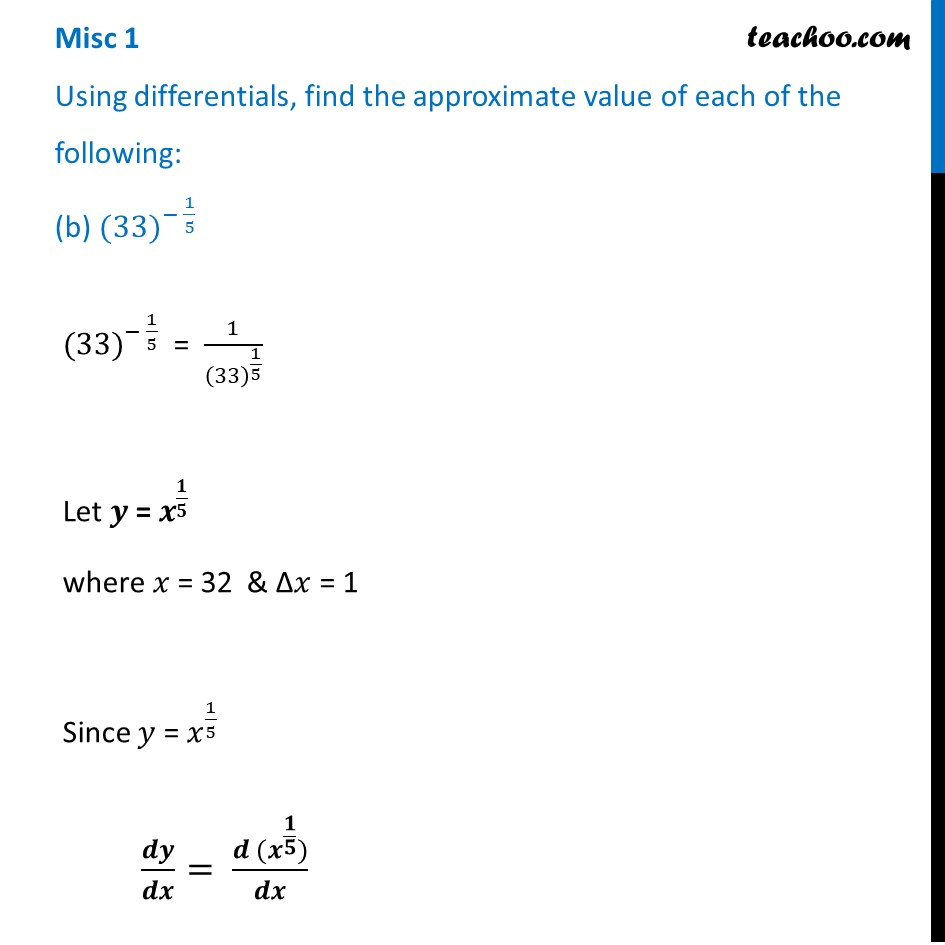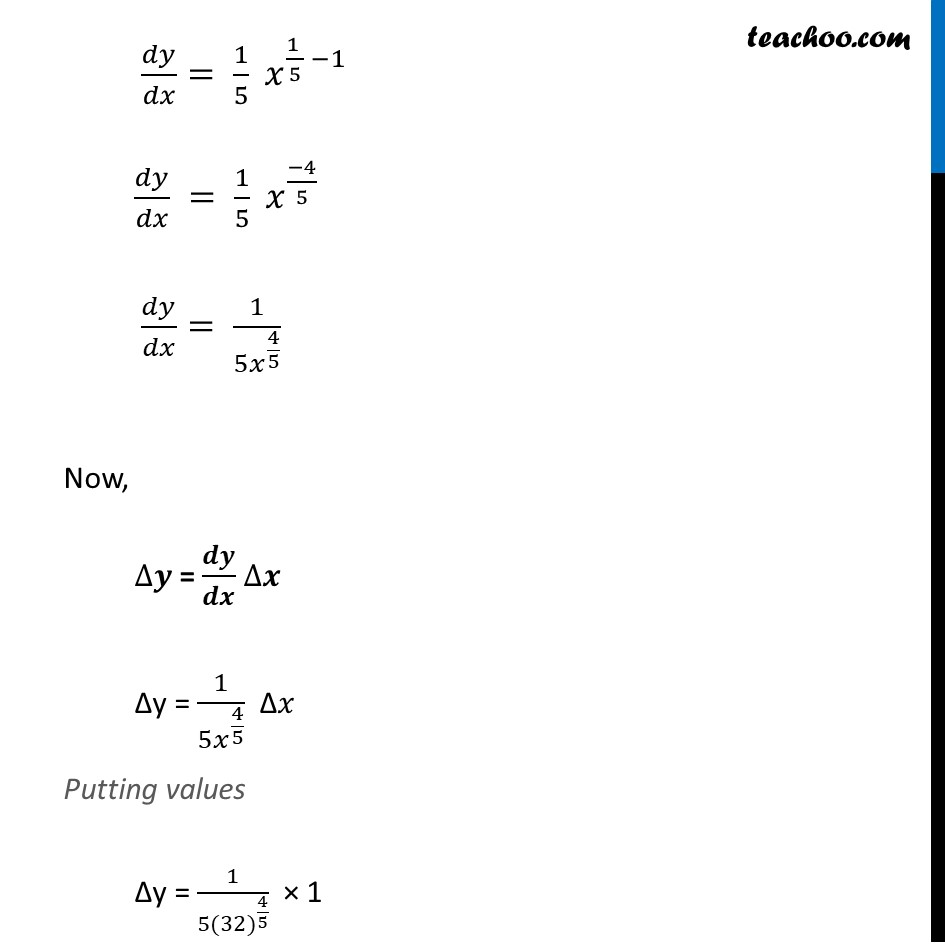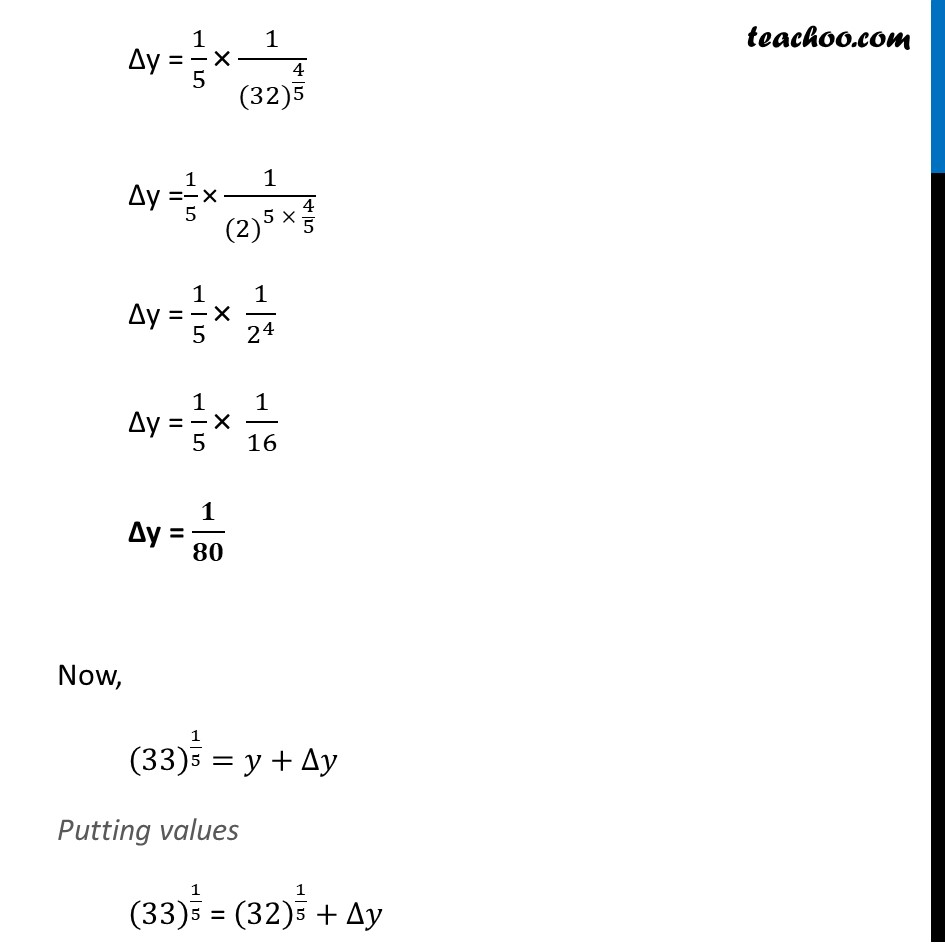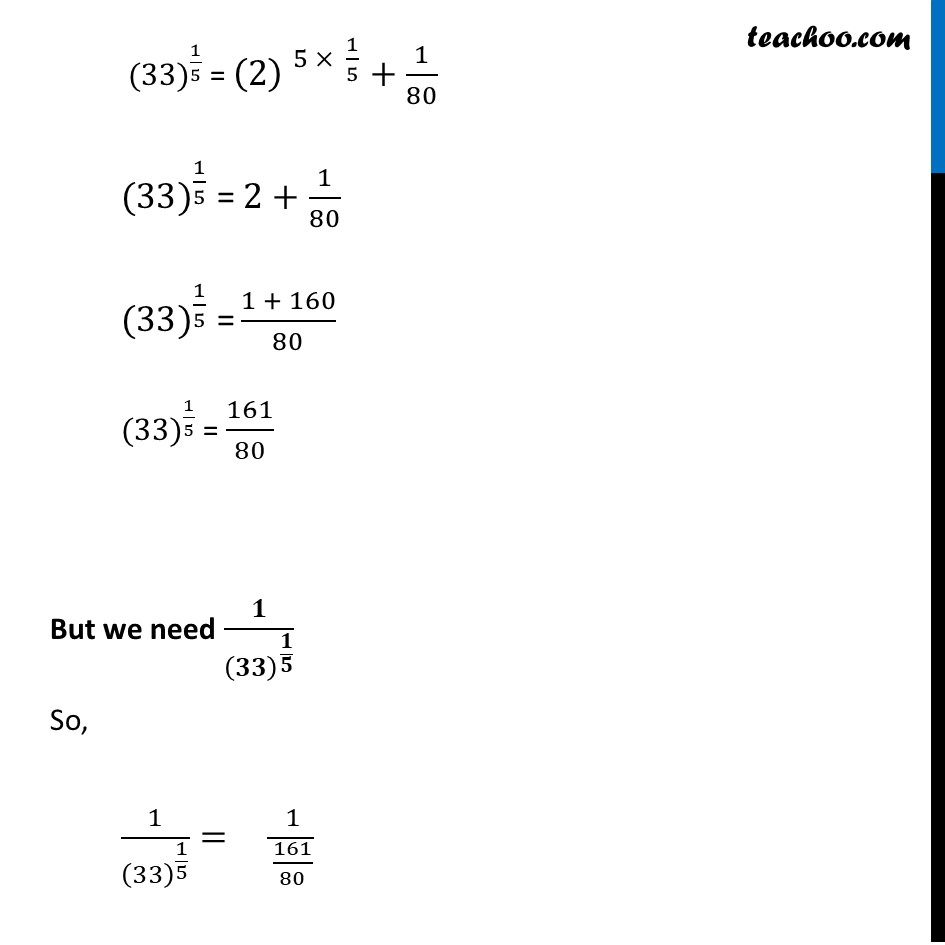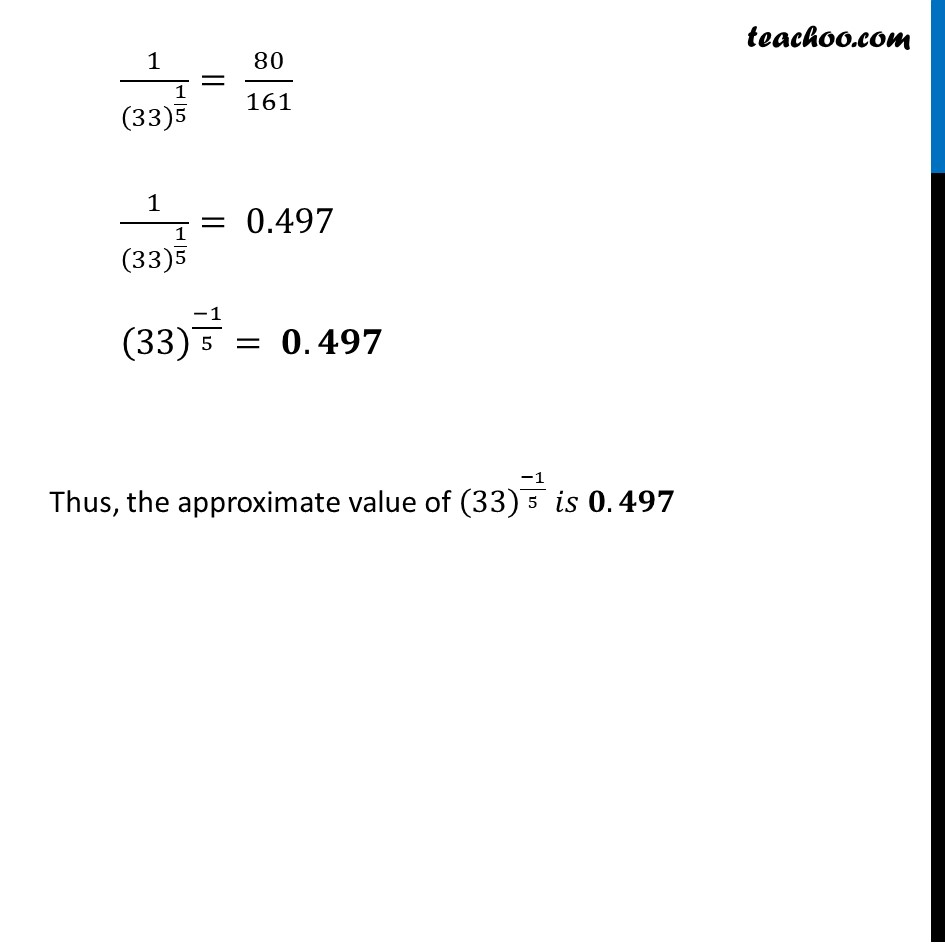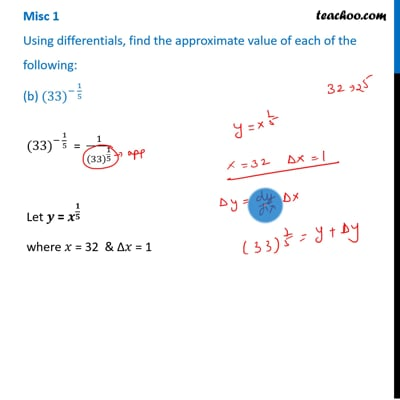This video is only available for Teachoo black users

Learn in your speed, with individual attention - Teachoo Maths 1-on-1 Class

### Transcript

Misc 1 Using differentials, find the approximate value of each of the following: (b) 〖(33)〗^(− 1/5)〖(33)〗^(− 1/5) = 1/(33)^(1/5) Let 𝒚 = 𝒙^(𝟏/𝟓) where 𝑥 = 32 & ∆𝑥 = 1 Since 𝑦 = 𝑥^(1/5) 𝒅𝒚/𝒅𝒙= (𝒅 (𝒙^(𝟏/𝟓)))/𝒅𝒙 𝑑𝑦/𝑑𝑥= 1/5 𝑥^((1 )/5 −1) 𝑑𝑦/𝑑𝑥 = 1/5 𝑥^((−4)/5) 𝑑𝑦/𝑑𝑥= 1/〖5𝑥〗^(4/5) Now, ∆𝒚 = 𝒅𝒚/𝒅𝒙 ∆𝒙 ∆y = 1/〖5𝑥〗^(4/5) ∆𝑥 Putting values ∆y = 1/〖5(32)〗^(4/5) × 1 ∆y = 1/5 "×" 1/〖(32)〗^(4/5) ∆y =1/5 "×" 1/〖(2)〗^(5 × 4/5) ∆y = 1/5 "×" 1/2^4 ∆y = 1/5 "×" 1/16 ∆y = 𝟏/𝟖𝟎 Now, (33)^(1/5)=𝑦+∆𝑦 Putting values (33)^(1/5) = (32)^(1/5)+∆𝑦 ∆y = 1/5 "×" 1/〖(32)〗^(4/5) ∆y =1/5 "×" 1/〖(2)〗^(5 × 4/5) ∆y = 1/5 "×" 1/2^4 ∆y = 1/5 "×" 1/16 ∆y = 𝟏/𝟖𝟎 Now, (33)^(1/5)=𝑦+∆𝑦 Putting values (33)^(1/5) = (32)^(1/5)+∆𝑦 〖(33)〗^(1/5) = 〖(2) 〗^(5 × 1/5)+1/80 〖(33)〗^(1/5) " =" 2+1/80 〖(33)〗^(1/5) " =" (1 + 160)/80 〖(33)〗^(1/5) = 161/80 But we need 𝟏/(𝟑𝟑)^(𝟏/𝟓) So, 1/(33)^(1/5) = 1/(161/80) 1/(33)^(1/5) = 80/161 1/(33)^(1/5) = 0.497 (33)^((−1)/5)= 𝟎.𝟒𝟗𝟕 Thus, the approximate value of (33)^((−1)/5) 𝑖𝑠 𝟎.𝟒𝟗𝟕